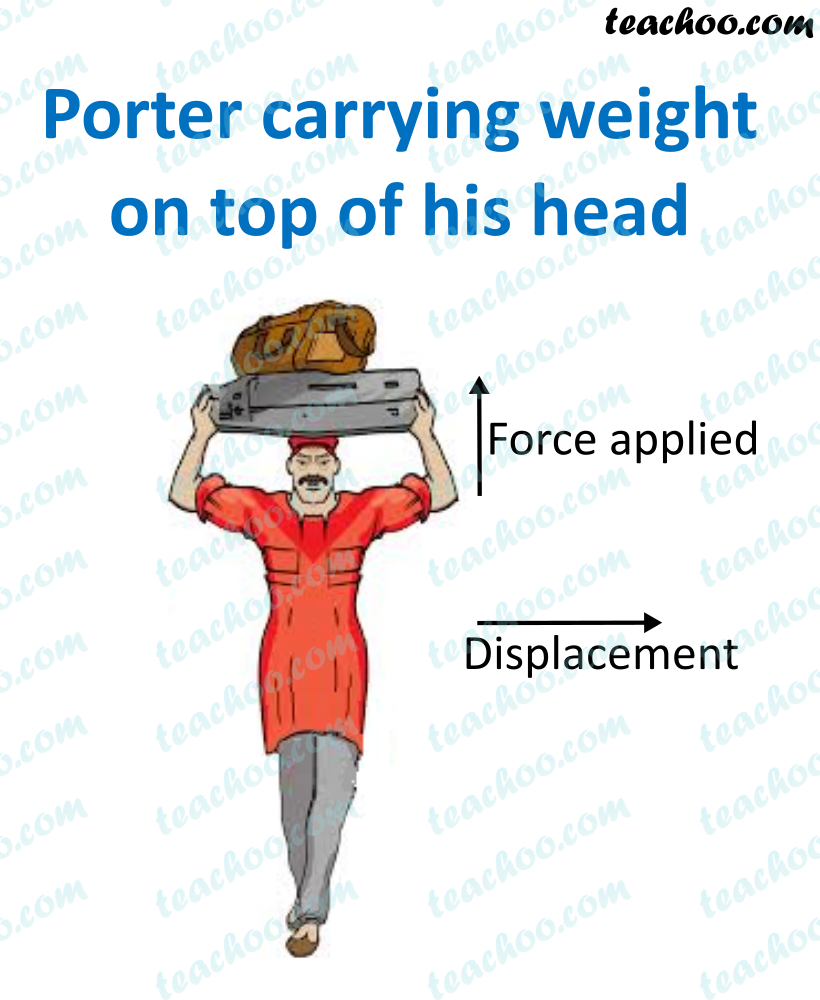Concepts

Class 9
Chapter 11 Class 9 - Work and Energy

## Positive Work

When force acts in direction of motion of body, it is called positive work

Example

Direction of force and Direction of Motion in the same direction

## Negative Work

When force acts opposite to direction of motion of body it is called negative work

Example

Direction of force and Direction of Motion are at angle of 180 degrees

## Zero Work

When force acts perpendicular to direction of body Or when there is no displacement

Example

• Direction of force and Direction of Motion are at right angle (angle of 90 degrees)
• Force is applied but there is no displacement

## When work done is Perpendicular to Direction of Force

Sometimes force acts perperndicular to the direction of body

So, angle made between force and distance is 90 degree

Thus, work done will be 0

### Explanation

We know that

Work = Force × Distance × cos θ

Work = Force × Distance × cos 90

Since cos 90 degree = 0

Work = Force × Distance × 0

Work = 0

Example

##googletag.cmd.push(function() { googletag.display('div-gpt-ad-1669298377854-0'); });

Here, Force is appled in vertical direction

But luggage is carried in horizontal direction

Since angle between force and distance is 90 degree

So, Work done is 0

Work Done when Force Acts opposite to Direction of Motion

When force acts opposite to direction of motion

Angle made between direction of force and direction of motion is 180 degree

In this case,

Work = Force × Distance × cos θ

Work = Force × Distance × cos 180

Since cos 180 degree = 0

Work = Force × Distance × -1

Work = - Force × Distance

So, in this case, negative work is done

## Questions

NCERT Question 2 - An object thrown at a certain angle to the ground moves in a curved path and falls back to the ground. The initial and the final points of the path of the object lie on the same horizontal line. What is the work done by the force of gravity on the object?

NCERT Question 5 - A mass of 10 kg is at a point A on a table. It is moved to a point B. If the line joining A and B is horizontal, what is the work done on the object by the gravitational force? Explain your answer.

NCERT Question 8 - Does the transfer of energy take place when you push a huge rock with all your might and fail to move it? Where is the energy you spend going?

Learn in your speed, with individual attention - Teachoo Maths 1-on-1 Class